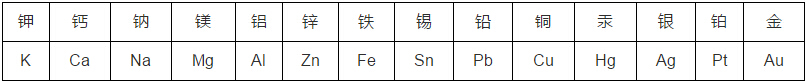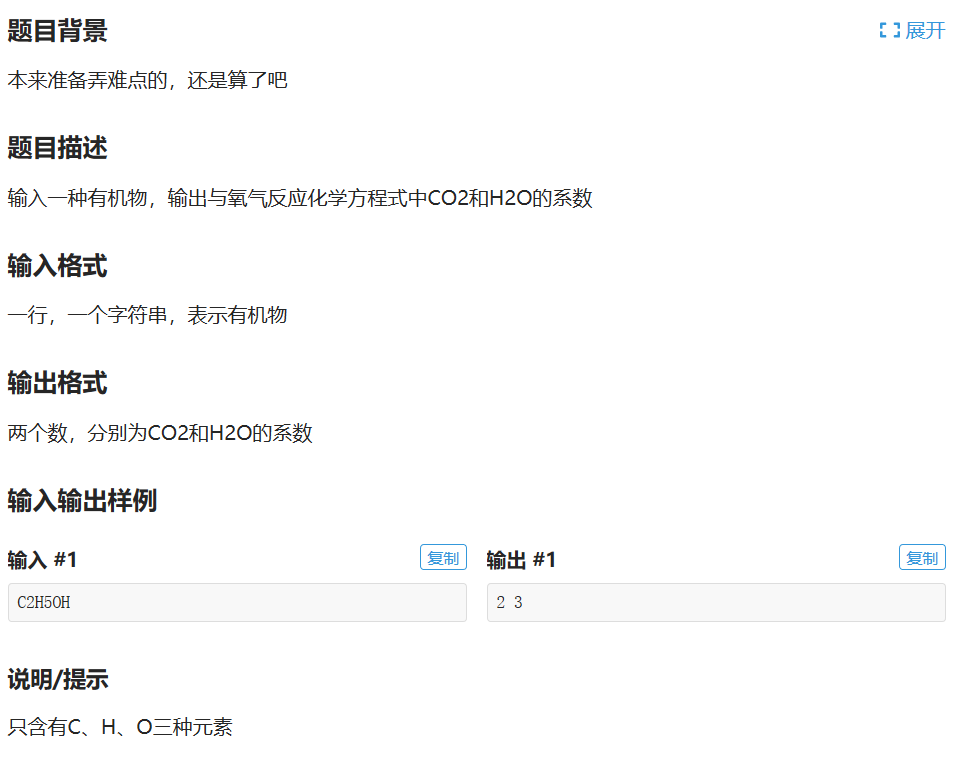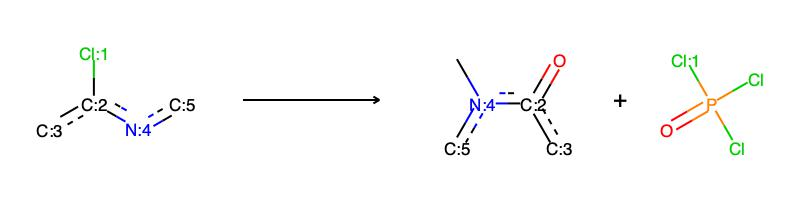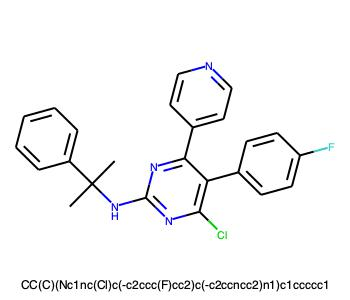• H2 O2 N2 Cl2 O3 I2 C Si S P 稀有气体： 氦气 氖气 氩气 化学式： He Ne Ar 氧化物： 氧化镁 氧化钙(生石灰)氧化铝 氧化铜 (黑色) 化学式： MgO CaO Al2O3 CuO 氧化铁(三氧化二铁,红色,铁锈主要成...
初中化学常见物质名称及化学式
金属单质：非金属单质：
氢气 氧气 氮气 氯气 臭氧 碘 碳 硅 硫 磷
化学式：
H2 O2 N2 Cl2 O3 I2 C Si S P
稀有气体：
氦气  氖气  氩气
化学式：
He   Ne   Ar
氧化物：
氧化镁 氧化钙(生石灰)氧化铝 氧化铜 (黑色)
化学式：
MgO   CaO   Al2O3    CuO
氧化铁(三氧化二铁,红色,铁锈主要成分)四氧化三铁(黑色) 二氧化锰(黑色，可做制氧催化剂)
化学式：
Fe2O3    Fe3O4   MnO2
水 双氧水(过氧化氢) 二氧化碳(温室效应) 一氧化碳(剧毒) 二氧化硫(酸雨) 五氧化二磷
化学式：
H2O  H2O2   CO2   CO   SO2   P2O5
酸：
盐酸 硫酸 碳酸 硝酸 亚硫酸
化学式：
HCl   H2SO4   H2CO3   HNO3   H2SO3
碱：
氢氧化钠(烧碱火碱苛性钠)氢氧化钙(熟石灰消石灰改良酸性土壤) 氢氧化铜(蓝色絮状沉淀)
化学式：
NaOH   Ca(OH)2   Cu(OH)2
氢氧化镁(白色沉淀) 氢氧化铁(红褐色沉淀) 氢氧化钡 一水合氨
化学式：
Mg(OH)2   Fe(OH)3   Ba(OH)2   NH3·H2O
盐酸盐：
氯化钠(食盐) 氯化钾 氯化银(不溶于水和酸) 氯化钙 氯化铜(蓝色)氯化铁(黄色) 氯化亚铁(浅绿色)
化学式：
NaCl   KCl   AgCl   CaCl2   CuCl2  FeCl3   FeCl2
硝酸盐：
硝酸钠 硝酸钾 硝酸银 硝酸钡 硝酸铜(蓝色)
化学式：
NaNO3  KNO3  AgNO3  Ba(NO3)2   Cu(NO3)2
碳酸盐：
碳酸钠 碳酸钾 碳酸钙(白色沉淀，遇酸溶解放出气体) 碳酸钡(同CaCO3)
化学式：
Na2CO3   K2CO3   CaCO3   BaCO3
硫酸盐：
硫酸钠 硫酸钾 硫酸钡 硫酸钙 无水硫酸铜(白色,检验水) 五水硫酸铜(蓝色)
化学式：
Na2SO4   K2SO4  BaSO4  CaSO4  CuSO4  CuSO4·5H2O
其他盐：
氯酸钾 锰酸钾 碳酸氢钠(易溶于水，与酸反应放出气体)
化学式：
KClO3  K2MnO4   NaHCO3
高锰酸钾：KMnO4
(紫黑色,溶于水得到紫色溶液,用于检验CO实验现象是紫色褪去)


展开全文• 有机物燃烧的化学方程式配平（洛谷P1994题题解，Java语言描述）
题目要求
P1994题目链接分析
我太菜了，亏得我高中时期还虐（or被虐）生化，如今多年过去竟然没分析出来坑点，我太菜了。
我开始盲目认为“元素守恒”，所以对$H$原子数求和，
$C_xH_yO_z$与氧气反应的化学方程式：
$C_xH_yO_z+(x+\frac{y}{4}-\frac{z}{2})+O_2→xCO_2+\frac{y}{2} H_2O$

$\frac{y}{2}$要是个整数，所以若$y$是个奇数，那就要$\times2$。
$(x+\frac{y}{4}-\frac{z}{2})$是个整数，因为$x$是个整数，所以要求$(\frac{y}{4}-\frac{z}{2})$是个整数，若此式不为整数，则继续$\times2$，即可配平为全整数系数。

AC代码（Java语言描述）
import java.util.Scanner;

public class Main {

private static int i = 0, c_counter = 0, h_counter = 0, o_counter = 0, temp = 0;

private static char[] formula;

private static int count() {
int counter = 0;
while (++i < formula.length && Character.isDigit(formula[i])) {
temp *= 10;
temp += formula[i]-'0';
}
if (temp == 0) {
counter++;
} else {
counter += temp;
temp = 0;
}
return counter;
}

public static void main(String[] args) {
Scanner scanner = new Scanner(System.in);
formula = scanner.nextLine().toCharArray();
scanner.close();
for ( ; i < formula.length; ) {
switch (formula[i]) {
case 'C':
c_counter += count();
break;
case 'H':
h_counter += count();
break;
default:
o_counter += count();
}
}
while ((h_counter & 1) != 0 || (h_counter - 2*o_counter) % 4 != 0) {
c_counter <<= 1;
h_counter <<= 1;
o_counter <<= 1;
}
System.out.println(c_counter + " " + h_counter/2);
}

}



展开全文字符串 algorithm 算法 java
• 物理化学笔记（1）量子化学基础 化学是人类关于原子和分子的知识和智慧的结晶。一个优秀的化学家需要适当了解其他科学分支的观测角度，在一定程度上听得懂其他学者的语言。但更重要和根本的是化学家一定要能流利地...
物理化学笔记（1）量子化学基础

化学是人类关于原子和分子的知识和智慧的结晶。一个优秀的化学家需要适当了解其他科学分支的观测角度，在一定程度上听得懂其他学者的语言。但更重要和根本的是化学家一定要能流利地使用分子语言。
脱离了量子化学的物理化学将变成完全宏观唯象的，二十世纪初的物理化学。如果学习完物理化学而不懂得联系宏观与原子分子，那样的物理化学无疑是一个遗憾

一、 薛定谔方程
1. 薛定谔方程基本表达
到目前为止，对单个原子、离子、分子的图像，最好的描述方法是量子力学。而对于这些具有波粒二象性的微观粒子，通过解薛定谔方程获取粒子波函数之后，微观体系的状态和该状态所决定的各种物理性质可用此波函数来表示，我们就可以得到体系内粒子的全部信息——这对于多粒子体系也是如此。对一维体系，薛定谔方程的形式如（1）式，而其三维空间的形式可以方便地利用梯度概念扩展，即（2）式：
$i\hbar\frac{\partial \Psi}{\partial t} = \frac{\hbar^2}{2m}\frac{\partial^2 \Psi}{\partial x^2} + V\Psi$
$i\hbar\frac{\partial \Psi}{\partial t} = \frac{\hbar^2}{2m} \nabla^2{\Psi} + V\Psi$
上式的$\Psi(x,t)$即为微观粒子体系的时空函数——波函数，它最关键的物理意义由Born的统计诠释指出，即$|\Psi(x,t)|^2$表示在t时刻在x处此粒子出现的概率密度，用公式表达为：
$\int_V |\Psi(x,y,z,t)|^2dxdydz = \left\{\text{在t时刻发现粒子在某体积元处的概率}\right\}$
上式的三维坐标在简化到一维坐标时表达完全相同的本质。这样的统计诠释赋予波函数以现实意义，也使它必须具有单值、有限、连续、归一化的条件，哪怕是随时间演化时也是如此。
2. 由波函数导出力学性质
量子力学体系是一套完备的数学物理体系，其中有一些我们需要知道的结论：

宏观体系的力学量是微观量子体系的对应量的统计平均值（严格意义上说，是对含有相同体系的系综的重复测量平均），也即“期望值”，这些微观体系的力学量的统计平均遵循经典力学的基本定律。

宏观系统中统计”期望“的表达如下，其中$\rho(x)$为概率密度函数，从某种意义上来说可以代表系统的粒子分配函数（在总体足够大时的统计分配）：
$\left = \int{x\rho(x)}dx$
$\left = \int{f(x)\rho(x)dx}$
这种统计期望必然满足：（注意：没有写出积分区间的积分号默认为全空间）
$\int_{-\infty}^{\infty}\rho(x)dx = 1$
$\left< \sigma^2\right> = \int\left({x-\left}\right)^2\rho(x)dx = \left-\left^2$
(7)式即为此统计形式的方差计算方法，这种方差在统计热力学中用来表示实际量相对于统计平均的”涨落“，而在量子力学中可以表示物理量的不确定度。在量子力学中，概率密度函数为$|\Psi(x,t)|^2$，从而坐标量的期望值可以表达为：
$\left = \int x|\Psi(x,t)|^2dx$
波函数一般都是复数形式，其模的计算满足$|\Psi(x,t)|^2 = \Psi\Psi^*$，从而坐标的期望值表达为：
$\left = \int \Psi x\Psi^*dx$

由于力学量期望值遵循经典力学定律，可以导出动量的期望值的表达：
$\left = m\frac{d\left}{dt}=-i\hbar\int\left(\Psi^* \frac{\partial \Psi}{\partial x} \right)dx = \int \Psi^*\left(-i\hbar\frac{\partial}{\partial x}\right)\Psi dx$
由此，引入动量算符$\hat p = -i\hbar\nabla$（一维的表达即如式10）和坐标算符$\hat x$（高维完全一样），由于经典力学中所有的力学量均表示为坐标和动量的函数，记为$Q(x,p)$，则每一个力学量对应一个算符$\hat Q(\hat x,\hat p)$，其期望值的求解只需要用$\hat p = -i\hbar\nabla$来取代$Q(x,p)$中的每一个$p$，然后将得到的算符放在$\Psi^*$和$\Psi$之间，再在空间中积分：
$\left = \int \Psi^*\hat Q(\hat x, \hat p)\Psi dx$
例如，动能的期望值为：
$\left = -\frac{\hbar^2}{2m}\int\Psi^*\frac{\partial^2 \Psi}{\partial x^2}dx$
对应于动能的算符为
$\hat T =-\frac{\hbar^2}{2m}\nabla^2$

薛定谔方程中的势函数$V(x,t)$也可以用算符来表达，一般就用$\hat V$表示

一旦波函数确定，整个体系的各个力学量期待值也就确定了，并且这些力学量期望值遵循经典力学定律，所有期望值均可由坐标和动量唯一确定——这也是对应性原理（Corresponding Principle）的必然要求。量子与计算化学的任务说到底就是发展各式各样的方法来求解薛定谔方程并得到体系的性质。

3.不确定性原理
对于任何波动现象，其波动的周期性越好，波长定义就越清晰，但波的具体位置就越不清晰，反之，如果波的具体位置越清晰，波动的周期性就越差，波长的定义就越不清晰，这一点可以从傅里叶(Fourier)分析中的一个定理严格证明，在定性的讨论中，综合波粒二象性粒子的德布罗意(de Broglie)关系：
$p = \frac{h}{\lambda} = \frac{2\pi\hbar}{\lambda}$
这样，波长的弥散就对应了动量的弥散，从而，对一个全同体系（系综）的测量不会产生同样的结果，粒子的位置定的越精确，动量就越不精确。通过量子力学的方法可以严格证明：
$\sigma_x \sigma_p \ge \frac{\hbar}{2}$
这就是著名的海森堡不确定性原理(Heisenberg’s Uncertainty Principle)，这也是微观粒子最重要的特征之一，即某些两两成对的性质，比如位置和动量、时间和能量、都不能同时确定。这不是仪器精准度的问题，而是波粒二象性的必然。
需要注意的是，一切物质都具有波粒二象性，从而不确定性原理对宏观和微观的一切物质均成立，只是位置和动量的弥散在宏观尺度上可以忽略不计。一切问题都有其本征精确度，如果我们一味追求精确而不做任何近似，那样的做法是愚蠢的。
4. 化学体系中的薛定谔方程
化学体系中，势函数$V(x,t)$由粒子间的作用形成，一般来说是不依赖时间的，在这种情况下通过分离变量法得到的薛定谔方程即为定态(time-independent)薛定谔方程：
$-\frac{\hbar^2}{2m}\nabla^2\psi+V\psi = E\psi$
或者表示为哈密顿算符的模式，式中E直接表达体系能量：
$\hat{H}\psi = E\psi$
$\psi(x)$即为粒子的定态波函数，关于这个“定态”的意义以及之后延伸出来的一系列量子模型将在后续讨论。需要指出的是，所谓“算符”就是一直用运算符号，它作用于一个函数，对这个函数施行算符所包含的数学运算。在量子力学中，每个厄米算符对于一个物理量，算符作用于其本征函数可以读出其对应物理量的本征值。后续的讨论中我们会发现，算符可以看做是波函数张成的Hilbert空间的子空间中的一个变换（一个变换是从自身集合到自身集合的映射）。
二、定态与定态波函数
我们已经给出了定态薛定谔方程，即式（17），接下来来讨论定态的性质。定态薛定谔方程是由非含时势函数结合分离变量法（这是物理学家解任何偏微分方程的首选）来将时间和坐标分开从而求解的，这个定态有以下特点

它们是定态(stationary states)，尽管波函数本身：
$\Psi(x,t) = \psi(x)e^{-iEt/\hbar}$
是与时间有关的，但是概率密度
$|\Psi(x,t)|^2 = \Psi\Psi^* = \psi^*e^{+iEt/\hbar}\psi e^{-iEt/\hbar} = \psi^*\psi = |\psi(x)|^2$
中的时间因子相互抵消，它就不依赖时间了。这一点可以推广到计算任何动力学变量的期望值，即（11）式变为：
$\left = \int\psi^*\hat Q(\hat x,\hat p)\psi dx$
推广到三维空间即：
$\left = \int_V\psi^*\hat Q(\hat x,\hat y,\hat z,\hat p)\psi dv$
任何一个力学期望值都是不依赖时间的，从而在很大程度上我们可以完全去掉时间因子$\varphi(t) = e^{-iEt/\hbar}$，用定态波函数$\psi$代替波函数$\Psi$，容易证明这个$\psi$也满足单值有限连续归一化的条件。但一定要明确真正的波函数是含时的。此后，我们提到的”波函数“一般均指定态波函数。
顺带一提，在定态中，$\left$必定为一常数，因此一定有$\left = 0$。

它们是具有确定总能量的态。（这点可以理解：定态的总能量确定而其时间是难以确定的），在严密的经典力学（哈密顿力学）中，总能量（动能加势能）称为哈密顿(Hamitonian)，
$H(x,p) = \frac{p^2}{2m}+V(x)$
对应的哈密顿算符也符合之前提到的替换规则：
$\hat H = -\frac{h^2}{2m}\nabla^2 + V(x)$
从而定态薛定谔方程可以由式（16）写成式（17）的形式。容易用上面的公式得出$\sigma_H^2 = 0$，也即对相同定态构成的系综的不同测量会得到相同的能量值，不存在弥散。
稍微一提，区分于牛顿力学的，严密的哈密顿力学以能量等作用量为基础，而力只是能量的负梯度，也即一阶Taylor展开系数。这里已经有了能量“是相互作用的标度”的概念，我们在统计热力学部分会进一步提及。

这样的定态中，一般解是分离变量解的线性叠加。含时薛定谔方程具有这样的性质：多个解函数$\Psi(x,y,z,t)$的线性叠加仍然是它的解（这点将在后续展开）。定态薛定谔方程（式17）给出一个无限的解集。一旦得到分离解，便可以立刻构造一个一般解，其形式为：
$\Psi(x,y,z,t) = \Sigma_{n=1}^{\infty}c_n\psi_n(x,y,z)e^{-iEt/\hbar}$

对于定态薛定谔方程的每一个归一化的解，其能量E必然要大于$V(x)$的最小值。否则，定态波函数将不可归一化，也就没有了意义。

三、波函数与希尔伯特空间
1. 定态波函数的正交归一性和完备性
在第二节中我们给出了定态薛定谔方程的形式及其所解得的定态波函数的一些特点。在实际求解具体体系的定态波函数并得到能量表达之前，让我们先来考察一下定态波函数的两个更有趣的性质，这两个性质在量子力学框架下业已严格证明：
1. 相同体系解出来的定态波函数是相互正交归一的。我们可以用狄克罗内克符号$\delta$来描述这个性质，这个特殊符号的定义如式（25）所示：
$\delta_{mn} \overset{\text{def}}{=} \begin{cases} 0&\text{m \neq n} \\ 1&\text{m = n} \end{cases}$
从而定态波函数的正交归一性表示如下：
$\int\psi_m(x)^*\psi_n(x)dx = \delta_{mn}$
2.相同体系解出来的波函数是完备的：也就是说任意一个函数$f(x)$都可以用这些定态波函数的线性叠加来表示，即：
$\forall x \in R, f(x) = \overset{\infty}{\underset{n=1}{\Sigma}} c_n\psi_n(x)$
式（27）实际上就是函数$f(x)$的Fourier展开式，傅里叶展开的原理告诉我们，任何一个函数均可以用一套周期内两两正交且完备的三角函数基底的级数展开式表达，这个基底为$\{cos(nx),sin(nx)|n\in N\}$，其展开式为：
$f(x) = a_0 + \overset{\infty}{\underset{n=1}{\Sigma}}\left(a_ncos(nx)+bnsin(nx)\right)$
此展开式表达$f(x)$的程度由狄利克雷(Dirichlet)定理可以说明：如果：$f(x)$在单个周期内除了有限个点外有意义并且单值，且$f(x)$和$f'(x)$在单个周期内分段光滑，那么在x的连续点上，Fourier展开式收敛于$f(x)$，而在x的间断点，Fourier展开式收敛于其左右极限的均值，即$\frac{(f(x+0)+f(x-0)}{2}$。利用基底正交的性质可以求得$a_0,a_n,b_n$，具体过程可以参照任意一本高等数学教材
回过头来看，定态波函数会具有这种级数展开的性质一点都不奇怪。根据欧拉公式$e^{ix} = cos(x) + isin(x)$，波函数无论是用三角函数形式表达还是用复指数形式表达，它们的本质应当是一致的。值得一提的是，基于Fourier展开的Fourier Transformation在量子力学，尤其是波动力学中，具有非常重要的应用，此处不再展开。
2.Hilbert空间：量子力学中的线性代数
从线性代数的角度来看，定态薛定谔方程，即式（17），和线性代数中的特征方程是一样的形式：
$\hat Ax = \lambda x$
如果细心观察，你会发现矩阵乘法其实就是一种线性变换，这种变换作用于特征向量(eigenvector)，即可以得到其特征值(eigenvalue)，而在式（17）中，力学量算符（即哈密顿算符）作用于其本征函数(eigenfunction)，可以得到对应的力学量的本征值(eigenvalue)，即能量。本征值和本征函数是国内结构化学与量子化学教材中常见的翻译，这里给出了英文原文，可以看出定态薛定谔方程和特征方程完全就是一回事。
事实上，对于一切算符，若将一个算符作用在一个函数$f$上，满足:
$\hat Gf = Gf$
则称$f$是算符$\hat G$的本征函数，G为$\hat G$的本征值。式（30）即是本征值方程。在量子力学中，所有力学量（能量，角动量等）均可用坐标和动量表达，它们对应的算符就是将坐标和动量的算符代入即可得到，对此波函数所表达的微观体系的状态，该力学量的测量所得到的结果就是对应力学量算符的本征值。
线性空间的严格定义可以参照任何一本高等代数教材，在广泛的线性空间之中，空间的基本元素——向量，不再局限于几何中的向量形式，而是在给定集合和数域内满足可加性和数乘比例性的任意抽象向量元。其中最经典的元素就是函数。自然科学中的函数实际上是数域到数域的映射，而只要选定一组基底，所有的函数均可以表示成这组基的线性表出的形式。显然，无论是Taylor展开还是Fourier展开，其本质都是选定一组基去线性表出所有的函数。
我们已经说明了给定体系波函数的正交归一性和完备性，这自然表明了波函数满足抽象向量的定义条件，与此同时，在量子力学框架内，力学量对应的算符作为线性变换作用于“向量”之上，因此，量子力学的自然语言是线性代数。
量子力学的“向量”是波函数，它们存在于无穷维线性空间中，除了类似于有限维线性空间（即欧几里得空间）的约束条件以外，为了表示可能的物理状态，波函数$\psi$必须是归一化的，因此这个空间实质上是所有在特定区域内平方可积函数的集合：
$\forall f(x), \int|f(x)|^2dx < \infty$
所构成的无限维线性空间。物理学家把这样的矢量空间即称作希尔伯特(Hilbert)空间，因此，在量子力学中，（含时）波函数是处于Hilbert空间之中，或者说，特定量子体系的所有解波函数张成一个Hilbert空间。需要指出的是，在严格的数学领域中，这个"Hilbert空间"只是严格意义上的希尔伯特空间的子空间。相应的，力学量算符则是Hilbert空间内的线性变换。
3.态叠加原理，内积和厄米算符
1. 内积和函数正交，归一，完备性
我们定义两个函数$f(x)$和$g(x)$的内积如下：
$\left = \int f(x)^*g(x)dx$
如果$f(x)$和$g(x)$都是平方可积的，即两者都在Hilbert空间之中，则它们的内积一定存在。我们可以从定义出发得到内积的两个基本性质：
$\left = \left^*$
$\left = \int|f(x)|^2dx = C (C \in R, C \ge 0)$
我们之前已经说明过函数的正交归一性和完备性的性质，此处用内积形式再次说明：
如果一个函数与自身的内积为1，我们称之为归一化的；如果两个函数的内积为0，那么这两个函数是正交的。如果一组函数既是归一的也是相互正交的，称它们为正交归一的。即：
$\left = \delta_{mn}$
以及，在Hilbert空间中，如果存在一组函数，其它任意函数都可以表示为这组函数的线性叠加，那么这组函数是完备的，即：
$f(x) =\overset{\infty}{\underset{n=1}{\Sigma}}c_nf_n(x)$
且如果函数是正交归一的，上式中的常数可以用Fourier展开的方法得到，用内积表示为：
$c_n =\left$
在（四）中我们会发现，一维无限深方势井的定态在*(0,a)*区间构成了一个完备正交归一系，而谐振子的定态则在$(-\infty,+\infty)$区间构成了一个完备正交归一系。
2. 态叠加原理
Hilbert空间中，所有可观测量算符的本征波函数是完备的，其相应的本征态所张成的线性空间也一定是完备的。即，对于Hilbert空间中的任意一个量子客体$\psi$，我们都可以把它写成所有本征函数的线性组合。
$\psi = {\Sigma}_n{C_i\psi_i}$
这就是所谓的态叠加原理。将它翻译为易于理解的语言便是：若$\psi_1,\psi2,...,\psi_n$为一微观体系可能状态的波函数，则由它们线性组合所得的$\psi$也是该体系可能存在的状态。这个$c_n$可以说明"$\Psi$中$\psi_n$的权重"，且对这些本征波函数而言，$|c_n|^2$是对一整套体系测量得到$E_n$的概率。
这种态叠加原理是分子轨道(MO)理论中轨道叠加组合的基础。
3.厄米算符与本征函数
一个力学可观测量$Q(x,p)$的期望值可以用内积符号简洁地表示出来：
$\left = \int \psi^*\hat Q\psi dx = \left<\psi|\hat Q \psi \right>$
一次测量的结果应当是实数，否则没有意义。这样一来多次测量的平均值也应是如此（注意，多次测量应当是对系综而言）
$\left = \left$
但由内积的性质，内积的复共轭会颠倒次序，因此：
$\left<\psi|\hat Q \psi \right> = \left<\hat Q \psi | \psi \right>$
Hilbert空间中的任意波函数都满足式（41），因此表示可观测量的算符有非常特殊的意义。我们给出如下定义：若算符$\hat Q$满足：
$\left = \left<\hat Qf|f \right>$
我们称这样的算符为厄米(hermitian)算符。即：可观测量由厄米算符表示，在《结构化学基础》上，它又被称作自轭算符。
厄米算符的定义表明它可以作用于内积的左侧项和右侧项且结果都一样。由于厄米算符的期望值是实数，它们自然出现在量子力学之中。且，由于力学量对应的算符在Hilbert空间内是线性变换，从而厄米算符也是线性的。
对于厄米算符的讨论存在离散谱和连续谱两种情况，我们需要掌握的是：

厄米算符表示一切可观测量算符

在离散谱中，厄米算符对可归一化的本征函数，其本征值必然是实数，并且属于不同本征值的本征函数相互正交。在连续谱中，内积可能不存在，需要另外讨论。

进一步推广到连续谱，有如下公理：厄米算符（可观测量算符）的本征函数是完备的，在Hilbert空间中，任何函数都可以用它们的线性叠加来表达

**广义统计诠释：**如果测量一个处于$\Psi(x.t)$态的粒子的可观测量$Q(x,p)$，那么其结果一定是厄米算符$\hat Q(\hat x, \hat p)$的一个本征值。
广义统计诠释将Born的只描述位形空间的波函数的统计意义扩展到了全部可观测力学量，即可观测力学量对应的本征函数的概率同样是该力学量空间下波函数模的平方，同时各个力学量空间的关系由傅里叶变换保证。而傅里叶变换的目的也正好是通过积分变换的形式进行空间的变换，因此数学又一次优雅的将不同物理现象统一了起来。

对于某个微观体系而言，如果描述该体系的波函数是某物理量$Q(x,p)$的厄米算符$\hat Q(\hat x,\hat p)$的本征函数，那么物理量$Q$的值即为本征值就和实验测定值相对应。若此波函数可以展开成Hilbert空间内一组波函数的线性展开
$\psi = {\Sigma}_n{C_i\psi_i}$
则物理量$Q(x,p)$的平均值（期望值）为：
$\left = \Sigma_n|c_n|^2Q_n$
如果描述该体系的波函数不是某物理量$Q$的本征态，我们可以用之前提到过的通用方法，用积分计算这个物理量的期望值，也即式（39）。

四、定态单，双粒子体系的处理
之前所讨论的都是为了建立一个相对完整的，基于薛定谔方程的量子力学图像，接下来我们来具体看看薛定谔方程在特定图景下的应用。
1. 势箱与平动能级
1.一维无限深方势阱
假设一个微观粒子在这样一个势场中：
$V(x) {=} \begin{cases} 0&\text{0 \le x \le a } \\ \infty&\text{anywhere else} \end{cases}$
一个粒子在这样的势能中除了在两个端点以外都是自由的，在端点处有无穷大的力限制它逃逸。国内教材往往把“势阱”译作“势箱”，其本质一致。图1. 一维无限深方势井示意图
在势阱外，$\psi(x)=0$，因为找到粒子的概率必然为零。在势井内，$V=0$，定态薛定谔方程可以写成如下的形式：
$-\frac{\hbar^2}{2m}\frac{d^2\psi}{dx^2} = E\psi$
或者写成：
$\frac{d^2\psi}{dx^2} = -k^2\psi, \text{ 其中 } k = \frac{\sqrt{2mE}}{\hbar}$
这是一个典型的描述谐振子运动的二阶微分方程，其一般解为：
$\psi(x) = Asinkx+Bcoskx$
这里的A和B属于积分常数，需要由问题的边界条件决定，这个边界条件一般来自于波函数的连续、有限、单值、归一化条件（在非端点处，归一化要求。波函数的连续性要求：
$\psi(0) =\psi(a)=0$
以使势阱内外解连续（这点其实和波尔氢原子论中的“定态驻波假定”是一样的），从而：
$\psi(0) = Asin0+Bcos0 = B = 0$
$\psi(a) = Asin(ka) = 0$
这样的边界条件不仅确定了波函数的形式，且确定了$k$的离散性：显然，式（49）中$A\neq0$，不然波函数$\psi(x)=0$，是不可归一化的无意义解。从而$sin(ka)=0$，这也就意味着：
$ka=n\pi, n\in N$
但是$k=0$没有意义，它也会得到$\psi(x)=0$的无意义解，而且负的解不给出新解，这源于正弦函数的奇函数性质。从而经过合并，可区分的解为：
$k_a = \frac{n\pi}{a}, n\in N_+$
这非常奇妙，在$x=a$处的边界条件没有确定常数A，却确定了常数$k$，因此我们得到了能量的表达式，即式（54）
顺带一提，这个$k$看似只是为了将微分方程转化为易于求解的形式，但它其实是具有物理意义的，在固体物理中这个$k$称作“波矢”，是K空间和能带的单位度量。
$E_n = \frac{\hbar^2k_n^2}{2m} = \frac{n^2h^2}{8ma^2}$
这和经典情况完全不同，一个量子化的粒子在一维无限深势阱中的能量不是任意的，它只是这些离散的特殊许可值。利用归一化条件我们可以求出波函数的系数的模：$|A|^2 = \frac{2}{a}$，对$A$我们可以简单地取其正根，从而势阱内波函数的解为：
$\psi(x) = \sqrt{\frac{2}{a}}sin\left(\frac{n\pi}{a}x\right)$
如前所述的，解定态薛定谔方程会得到一个无限的解集（每个正整数n对应一个解），一维势阱内的波函数的形式是长度在$a$的弦上的驻波，如图所示为此体系的前三个定态图2. 一维无限深方势井的前三个定态
不难发现，随着能量的增加，态的节点（与x轴的交点）数逐次增1，对于第$n$个量子态，其节点数目为$n-1$个。这一点对各类波函数的径向分布都是成立的。
在各个定态中，$\psi_!$具有最低的能量，即称为”基态“，其它态的能量正比于$n^2$增加，它们称为”激发态“。不难发现$E_1 \ne 0$，因此量子体系是存在零点能的。这表明了运动的永恒性，它也是不确定性原理的必然结果。
总的来说，由一维无限深势阱的波函数求解我们可以得到量子体系的如下特征：

粒子存在多种运动状态，它们可由$\psi_1,\psi_2, ... ,\psi_n$等描述
能量量子化
存在零点能
微观粒子没有经典的运动轨道，只有概率分布
波函数及其对应的粒子分布均存在节点，节点多的组态能量高。

2. 从势箱到平动能级
一维无限深势阱的结论可以直接扩展到三维，这和一维薛定谔方程扩展到三维的方法一模一样，加个正交坐标就好了。在长，宽，高为$l_x,l_y,l_z$的三维无限深势阱中，其薛定谔方程用式（2）表达，从而其波函数解为：
$\psi = \sqrt{\frac{8}{l_xl_yl_z}}sin(\frac{n_x\pi x}{l_x})sin(\frac{n_y\pi y}{l_y})sin(\frac{n_z\pi z}{l_z})$
式中$n_x,n_y,n_z$为三个方向自由度的“主量子数”，均为正整数。对应各波函数的能量表达式为：
$E_{xyz} = \frac{h^2}{8m}(\frac{n_x^2}{l_x^2}+\frac{n_y^2}{l_y^2}+\frac{n_z^2}{l_z^2})$
这就是微观粒子在三维（无限深）势阱中自由平动的能量表达式，对于分子、原子等质量相对较大，隧穿效应很不明显的微观粒子，可以用箱中粒子模型考虑它们的自由平动状态，认为粒子在碰到边界时会以完全弹性碰撞地形式被弹回，此时式（57）即为平动能级的表达式。
3. 量子隧穿效应简介
为确定平动能级能量表达式的适用范围，我们来简要考查一下量子隧穿效应。量子隧穿原理最简单的模型是一维方势垒，如图所示：图3. 一维方势垒示意图
在高度为U的势垒中，粒子的薛定谔方程如下
$-\frac{\hbar^2}{2m}\frac{d^2}{dx^2}\psi(x)+U\psi = E\psi$
当粒子能量$E>U$时，方程有如下解：
$\psi(x) = \psi(0)e^{\pm ikx},k=\sqrt{2m(E-U)}/\hbar$
上式$k$为波矢。而当粒子能量$E时，方程有如下解：
$\psi(x) = \psi(0)e^{- kx},k=\sqrt{2m(U-E)}/\hbar$
此时$k$为衰减因子，它描述电子波函数在水平方向$x$的衰减。不难发现，即便是势垒U大于粒子的能量E，微观粒子在这个势垒内依然有存在非零的出现概率，只是这个概率和波函数一样呈指数级衰减而已，其正比于$|\psi(0)|^2e^{-2kx}$。这就是量子隧穿效应，它表明微观粒子可以“渗透”穿过任何有限的势垒。
粒子穿过势垒的概率可以表示为：
$T = [1+\frac{1}{2}(\frac{k}{q}+\frac{q}{k})^2sinh^2(kW)]^{-1}$
其中$q = \sqrt{2mE}/\hbar$为粒子的初始波矢，W为势垒的轴向长度。其具体推导过程可以在任何一本量子力学书中找到。我们不难发现，若粒子的质量不是很小，在原子、分子级别，衰减因子$k$的值偏大，微观粒子的隧穿效应呈指数形式快速衰减，其穿过势垒的概率T很小，隧穿效应是不需要考虑的，我们可以用之前给出的一维无限深势阱来考虑其平动能级。
相应的，如果这个微观粒子是电子，其质量$m_e = 9.109\times10^{-31}g$，远小于原子和分子的质量（质子$m_p = 1.673\times10^{27}g$），它的隧穿效应就相对明显，在微观尺度上需要考虑，即金属表面的电子存在克服功函势垒向表面外“逸出”的运动形式。这就是扫描隧道显微镜（STM）的原理基础。利用量子力学方法可以导出，在金属表面-真空-金属针尖模型中，电子能够在所加偏压$V$远小于功函的情况下从金属表面隧穿到金属针尖形成隧道电流，但相应的，这样的隧道电流随针尖-样品的距离呈指数级衰减。具体原理可以查阅固体物理相关教材图4. 金属表面-真空-金属针尖的一维隧穿模型示意图
2. 一维谐振子模型与振动能级
经典谐振子的模型是一个质量为$m$的物体挂在一个力常数为$k$的弹簧上，其运动由胡克(Hooke)定律决定：
$F = -kx = m\frac{d^2x}{dt^2}$
此谐振子的振动情况遵循正弦波规律，忽略摩擦其解为：
$x(t) = Asin(wt)+Bcos(wt)$
其中 $w$为谐振子圆频率（角频率）
$w = \sqrt{\frac{k}{m}}$
如果是两个由弹簧连接的物体组成的模型，如双原子分子模型，用它们间的约化质量代替上式中的m即可，约化质量$\mu$的表达式为：
$\mu = \frac{m_1 \cdot m_2}{m_1+m_2}$
谐振子体系的势能为：
$V(x) = \frac{1}{2}kx^2 + V_0$
$V_0$为零点能，为积分常数，取决于边界条件。其$V-x$图是抛物线状的。
当然，没有完全理想的谐振子。如果弹簧伸长太多，胡克定律就会失效，弹簧甚至可能被破坏。但在实际中，任何势能在其极小值附近都可以用抛物线近似，如图所示：图5. 对任意势能极小值点附近的抛物线形（谐振子）近似
形式上，如果我们将势函数$V(x)$在极小值附近做Taylor展开：
$V(x) = V(x_0)+V'(x0)(x-x_0)_+\frac{1}{2}V''(x_0)(x-x_0)^2+...$
在极小值处$V’(x_0) = 0$，忽略高次项（只要$x-x_0$很小就可以忽略），得到：
$V(x) = V(x_0)+\frac{1}{2}V''(x_0)(x-x_0)^2$
这正是描述$x=x_0$附近处谐振子的势，其有效弹性常数$k = V''(x_0)$。事实上，任何振动形式，只要振幅足够小，都可以近似看做简谐振动，这就是谐振子为什么如此重要的原因。
量子力学的问题是要解势能为：
$V(x) = \frac{1}{2}w^2x^2$
时的定态薛定谔方程：
$-\frac{\hbar^2}{2m}\frac{d^2\psi}{dx^2}+\frac{1}{2}mw^2x^2 = E\psi$
这个方程的解法不同于势箱粒子，比较复杂，我们可以利用一种叫阶梯法的有趣方法，结合归一化方法解得谐振子模型的体系总能量的量子化能量表达（而波函数表达更加复杂）：
$E_n = \left(n+\frac{1}{2}\right)\hbar w = \left(n+\frac{1}{2}\right)h\nu$
具体解法可以参照任意一本量子物理教材。值得注意的是，谐振子模型中势能与位置有关，但总能量应当是守恒的，也即此模型的总能量中不仅存在势能项还存在动能项。如果代入波函数的表达，我们可以证明：
$\left = \frac{1}{2}\hbar w\left(n+\frac{1}{2}\right)$
可以看出，势能的期待值正好是总能量的一半，而另一半当然是动能。事实上，线性谐振子的一个自由度上同时存在动能项和势能项，这点在后续的讨论中会多次提到。
式（71）即为双原子分子的振动能级表达式，在很大程度上，作为一种分子简谐振动能级表达，它可以用于很多分子的各振动自由度上的的振动能级表达——因为各个振动自由度可以被近似认为定域在两个原子之间。进一步的精确表达需要对势函数做进一步的Taylor展开。图6. 分子势能曲线表示图
3. 转动能级
双原子分子的转动能级推导用的是刚性转子模型，由于转动体系是自由的，只有动能，没有势能，从而：
$E = T = \frac{M^2}{2I}$
其中$M$为角动量。量子力学证明体系的角动量是量子化的，其值为：
$M = \sqrt{J(J+1)}\frac{h}{2\pi}, J \in N$
从而转动能级的表达式为：
$E_J = J(J+1)\frac{h^2}{8\pi^2I}$
$J$可以称作分子的“总角量子数”，这个表达式可以利用统计热力学方法进行推广。需要注意的是，在量子力学刚性转子模型中，角动量不仅大小是量子化的，其方向也是量子化的，它用“总磁量子数“$S$来表示，量子力学可以证明每一个转动能级上磁量子数的大小可以为：$S_J = 0, \pm1, \pm2,...,\pm J$，由于取向不影响能量大小，转动能级的每一个能级的简并度即为$(2J+1)$，对应角动量的$(2J+1)$个取向。
4.分子运动形式与分子光谱简介
以上，我们将（双原子）分子的运动形式分成了平动（势箱模型），转动（刚性转子模型），振动（谐振子模型）三种运动形式，它们对应一个分子的三种能量形式。要将它们这几个分立的描述合起来描述一个分子的量子化行为，其必然要求是这几种运动形式是相互独立的，也即分子各个运动形式间应当具有独立性。
一个分子事实上还具有电子能级和核能级，它们表示构成分子的电子和原子核的能级状态（包括能量、轨道对称性、空间分布状况等），尤其是分子的电子能级，它决定了分子的主要性质。如果这几种运动形式不能相互独立，那么，即使我们能熟练运用量子力学方法，分子体系的处理也将变得十分困难
事实上，分子光谱实验的结果表明，各个不同的运动形式的能级差都分布在不同的数量级上，且各个运动形式的特征时间也分布在不同的数量级上。单独研究电子吸光的吸收光谱时，实验不但能重复地观察到固定的吸收峰，而且，只有在假定电子跃迁的同时原子核的位置不变的基础之上，电子光谱的一些精细结构才可以合理解释。这种定核近似的合理描述在光谱学中形成了著名的弗兰克-康登(Franck-Condon)原理。
大量光谱学实验结果和理论分析表明，（同核双原子）分子的波函数可以分成五种运动形式的波函数部分的乘积：
$\psi = \psi_t \cdot \psi_r \cdot\psi_v \cdot \psi_e \cdot \psi_N$
相对应的，分子的各种能级是量子化的，各种能级在一定条件下可以独立考虑，且各种运动形式耦合构成了整个分子的运动模式，即：
$\varepsilon_{total}=\varepsilon_{t}+\varepsilon_{r}+\varepsilon_{v}+\varepsilon_{e}+\varepsilon_{N}$
这就是分子各种运动的独立性和耦合性。上两式各项分别对应平动、转动、振动、电子、核五种运动形式，它们对应的分子光谱情况示意如下：图7. 分子光谱示意图 、

能级
能隙大小
激发难易

平动能级
~$10^{-18}eV, 10^{-19}kT$
极小

转动能级
$(0.05-10^{-4})eV$, ~$10^{-4}kT$
较小

振动能级
~$0.05eV, 10kT$
中等

电子能级
$(0.1 - 5)eV$
较难

核能级
$>10eV$
很难

表1. 各运动形式能隙示意
光谱(spectroscopy)是化学家观察世界和研究世界的眼睛，它们帮助化学家观察到原子分子尺度的分子运动，如果说晶体衍射打开了晶体研究的大门，STM打开了表面科学研究的大门，那么，原子分子光谱也就当之无愧地打开了一切原子分子层面的研究的大门。
五、量子化学的自底向上
无论是经典力学，还是量子力学，其基础都是偏微分方程，对于三个粒子以上的体系，其精确的解析解都是不存在的，从而，为了确实地解决多分子体系问题，我们有这样两条路可以走：
1. 计算化学：量子化学方法的进一步发展
1998年的诺贝尔奖授予了理论计算化学的两位奠基者Walter Kohn和John A. Pople，当时的颁奖词中如此写到：”化学已经不再是一门纯粹的实验科学“，今天，随着计算机科学和数学物理方法的快速发展，计算化学的理论和方法也迅猛成长着，开始展现出其强大的威力，现在的计算化学虽然还做不到“预测”的程度，但已经在化学研究中和实验化学并驾齐驱，互为补充。
理论计算化学并不能说是一门新兴的学科，上个世纪以来，从最初的Hartree-Fock自洽场，到密度泛函理论和Kohn-Sham方程，再到如今一套套相对成熟的计算化学软件包和计算化学方法，其核心都是一个——求解多体体系的薛定谔方程，进而得到体系的全部性质。这些理论在当时没有得到认可，很大一部分原因在于当时的算力远远达不到这些理论方法的要求。时至今日，算力虽然依然是一个限制因素，但我们已经可以在利用计算化学软件，基于量子化学和从头计算法研究简单体系化学问题的同时，进一步发展更好的量子化学计算方法，来探求更多更大体系的理论模拟。
理论模拟的可能并不代表我们能够抛弃实验，相反的——从实验中总结与感悟出来的那些化学的规律和直觉，往往是理论化学家区别于凝聚态物理和原子物理研究者的最大特色，这也是另一种层面上的”More is Different"。我们在用好计算机模拟方法的同时，一定不要忘了研究化学问题的初心，和综合化学理论的思维。
2. 从量子化学到统计热力学
化学所要处理的体系往往是多分子体系，而在粒子数多于$10^6$个的多分子体系之中，最概然分布已经占到宏观体系中所有微观状态分布的统治地位。从而我们可以用最概然分布的特征来描写宏观体系平衡态，这就是统计热力学的理论基础。基于此，导出了经典的麦克斯韦-玻尔兹曼（MB）分布和量子化的玻色-爱因斯坦（BE）分布以及费米-狄拉克（FD）分布。从经典的MB分布向上可以导出宏观经典热力学的许多结论。
事实上，结合量子化能级的经典MB分布已经可以自底向上地描述宏观热力学的微观图像，而总包了MB分布的宏观热力学作为“化学热力学”这门学科起着完美地多分子体系化学理论的作用。完善并运用好这套多分子体系理论，这应当是化学家们的努力方向。
参考书目：

《量子力学》格里菲斯
《彭笑刚 物理化学讲义》
《物质结构》徐光宪
《结构化学基础 第四版》


展开全文markdown
• 文章目录一、引入所需库二、化学反应实例三、化学反应模板四、化学反应注意事项 RDKit提供化学反应引擎，其中化学反应模板是基于smarts构建。反应物+反应引擎就可以生成产物。 一、引入所需库 #! /usr/bin/python # ...
文章目录一、引入所需库二、化学反应实例三、化学反应模板四、化学反应注意事项
RDKit提供化学反应引擎，其中化学反应模板是基于smarts构建。反应物+反应引擎就可以生成产物。
一、引入所需库
#! /usr/bin/python
# coding: utf-8

from rdkit import Chem

from rdkit.Chem import AllChem
from rdkit.Chem import Draw

二、化学反应实例
示例反应
rxn = AllChem.ReactionFromSmarts(
'([Cl;H0;D1;+0:1]-[c;H0;D3;+0:2](:[c:3]):[n;H0;D2;+0:4]:[c:5])>>(C-[n;H0;D3;+0:4](:[c:5]):[c;H0;D3;+0:2](=O):[c:3]).(Cl-P(-Cl)(=O)-[Cl;H0;D1;+0:1])')
img = Draw.ReactionToImage(
rxn
)
img.save(
'/Users/zeoy/st/drug_development/st_rdcit/img/mol31.jpg'
)

反应模板如下图所示：从反应模板中，我们看到主要的变化是Cl变成羰基氧，N上多了一个甲基

注：这是一个逆反应模板

反应物如下图所示 ：
mol = Chem.MolFromSmiles(
'CC(C)(Nc1nc(Cl)c(-c2ccc(F)cc2)c(-c2ccncc2)n1)c1ccccc1')
Draw.MolToImageFile(
mol,
"/Users/zeoy/st/drug_development/st_rdcit/img/mol32.jpg",
size=(350, 300),
legend='CC(C)(Nc1nc(Cl)c(-c2ccc(F)cc2)c(-c2ccncc2)n1)c1ccccc1'
). 创建具体反应规则的引擎对象rxn = AllChem.ReactionFromSmarts(tem)
.输入反应物，借助引擎产生反应rxn.RunReactants([productmol])
def getrxns(rxn, product_smi):
"""
获取反应规则的引擎对象
product_smi 反应物
"""
product_mol = Chem.MolFromSmiles(product_smi)
reactions = rxn.RunReactants([product_mol])
rxns = []
for reaction in reactions:
smis = []
for compound in reaction:
smi = Chem.MolToSmiles(compound)
smis.append(smi)

rxnstr = '.'.join(smis) + '>>' + product_smi
# newr = canon_reaction(rxnstr)
# rxns.append(newr)
return rxns

tem = '([Cl;H0;D1;+0:1]-[c;H0;D3;+0:2](:[c:3]):[n;H0;D2;+0:4]:[c:5])>>(C-[n;H0;D3;+0:4](:[c:5]):[c;H0;D3;+0:2](=O):[c:3]).(Cl-P(-Cl)(=O)-[Cl;H0;D1;+0:1])'
rxn = AllChem.ReactionFromSmarts(tem)
product_smi = 'CC(C)(Nc1nc(Cl)c(-c2ccc(F)cc2)c(-c2ccncc2)n1)c1ccccc1'
reactions = getrxns(rxn, product_smi)
for reaction in reactions:
img = Draw.ReactionStringToImage(reaction)
display(img)

三、化学反应模板
化学反应模板主要可通过两种方法获取：1. 自动抽取；2. 化学家编码。

对反应模板进行高亮显示 ：
化学家编码 化学家通过对反应进行归纳，整理出的反应规则，典型代表Chemtic 软件。

四、化学反应注意事项
利用化学反应引擎产生的产物，不一定符合化学规则，因此需要进行对产物进行检查。
tem = '([Cl;H0;D1;+0:1]-[c;H0;D3;+0:2](:[c:3]):[n;H0;D2;+0:4]:[c:5])>>(C-[n;H0;D3;+0:4](:[c:5]):[c;H0;D3;+0:2](=O):[c:3]).(Cl-P(-Cl)(=O)-[Cl;H0;D1;+0:1])'
rxn = AllChem.ReactionFromSmarts(tem)

def getrxns_reactants(rxn, product_smi):
product_mol = Chem.MolFromSmiles(product_smi)
reactions = rxn.RunReactants([product_mol])
rxns = []
for reaction in reactions:
smis = []
for compound in reaction:
smi = Chem.MolToSmiles(compound)
smis.append(smi)

newr = '.'.join(smis)
rxns.append(newr)
return rxns

prosmi = "COC(=O)c1cccc(-c2nc(Cl)cc3c(OC)nc(C(C)C)n23)c1"
rs = getrxns_reactants(rxn, prosmi)

print(rs)  # ['COC(=O)c1cccc(-c2n(C)c(=O)cc3c(OC)nc(C(C)C)n23)c1.O=P(Cl)(Cl)Cl']
print(len(rs))  # 1

smi = rs
m = Chem.MolFromSmiles(smi, sanitize=False)

if m is None:
print('invalid Smiles')
else:
try:
mi = Chem.SanitizeMol(m)
print(mi)
print('smiles is ok')
except:
print('invalid chemistry')  # invalid chemistry




展开全文rdkit 模板
• 土壤化学计量特性影响土壤碳、氮和磷循环微生物丰度及其对全球变化的抵抗力Soil Carbon, Nitrogen, and Phosphorus Cycling MicrobialPop...人工智能 机器学习 xhtml 深度学习 大数据
• 化学结构中的环对应于图论中的一个循环（更确切地说，这是一个简单的循环，因为它是一个循环不多次通过同一节点的循环）。可以通过跟随某个节点中的相邻节点来判断图是否具有循环。如果您可以从其他路线到达已经到达...生物信息学
• 将研究化合物之间的转化，即如何处理化学信息学中的化学反应。 首先，您将学习如何扩展单个化合物的表示法，例如先前处理的MOL文件和SMILES表示法，以表达化学反应。接下来，让学习如何在RDKit中处理化学反应。 ...Python
• 全世界只有3.14 %的人关注了爆炸吧知识化学的神奇魅力可是不是随便说说的，神奇起来让人叹为观止。下面37张动图，在带领你领略化学之美的同时，也希望能帮助你理解这些化学现象。1 . ...
• ## SMILES化学式解析

千次阅读 2019-01-16 13:41:23
化学式，所有的元素（键信息在元素中） package com . Demo2 ; import java . util . ArrayList ; public class ChemicalFormula { private ArrayList < ChemicalElement > Formula ; /** ...SMILES 符号解析
• Chainer Chemistry是一个使用Chainer的化学和生物学深度学习库。 Github地址：https://github.com/pfnet-research/chainer-chemistry 手册地址：https://chainer-chemistry.readthedocs.io 该库可帮助您轻松地将...深度学习 AI 生物信息学
• 1. CDK (Chemistry Development ...CDK是结构化学信息学和生物信息学的开源Java库。 该项目由Christoph Steinbeck，Egon Willighagen与Jmol和JChemPaint的开发人员Dan Gezelter于2000年发起。迄今为止，它是在科学...Python
• 1、【判断题】氧气的化学性质很活泼，在一定条件下自己也会燃烧。（）（×） 2、【判断题】气瓶钢印模糊不清、或难于辨认的，该气瓶应作报废处理。（√） 3、【判断题】依照气瓶的充装系数，是实施控制气瓶充装量...
• 化学方程式配平目录1 效果展示2 程序原理3 完整代码 1 效果展示 输入反应物、生成物后点击按钮即可配平（注意大小写）： 当前后所含元素不匹配，或者反应物生成物未填写时，会显示物质不会凭空出现或消失这句话。 ...matlab 整数规划
• 如三氟甲基（一种常用于药物发现化学中的部分结构）可以一步一步直接引入到药物分子本身中，是否有可能创造出一个具有新特性的有用分子？ 如果一口气就能制造出来，它们就能增加有前途的分子的种类！” 反应形式...RDKit
• 1483 化学变换 题目来源： CodeForces 基准时间限制：1 秒 空间限制：131072 KB 分值: 20 难度：3级算法题 收藏 关注 有n种不同的化学试剂。第i种有ai升。每次实验都要把所有的...
• 1、【判断题】氧气的化学性质很活泼，在一定条件下自己也会燃烧。（）（×） 2、【判断题】对于盛装可燃性气体和毒性气体的气瓶，在排除其瓶阀故障时，可以直接排放到大气中；（×） 3、【判断题】GB14193-...
• ## 原子化学势的计算

千次阅读 2020-04-10 21:58:48
• 【综述】计算化学的深度学习 全文基于谷歌翻译，语句多有不通顺 摘要 人工神经网络的兴衰在计算机科学和计算化学的科学文献中都有详细记载。然而近二十年后，我们现在看到了对深度学习兴趣的复兴，这是一种...
• 从TI官网上下载最新的化学ID更新的.ZIP包文件 　step2. 打开软件，点击help>Update Chemistry>选择相应的ZIP文件，更新即可。 　由于这里我已经更新过了，所以显示如下： 转载于:...
• 1．复习重点1．浓度,压强和温度等条件对化学平衡的影响.2．平衡移动原理的应用.2．...化学平衡的移动: 可逆反应中,旧化学平衡的破坏,新化学平衡的建立的过程就叫做化学平衡的移动.(一) 浓度对化学平衡的影响c
• 最近写论文需要画化学结构式，于是想到用Latex里的包。但是一看知乎里面的大牛们一片口诛笔伐，说还是Chemdraw好。用latex是装。。。 不管怎么说，还是查了一下。首先需要下载chemfig.tex，...
• ## 化学方程式配平器

千次阅读 2018-01-22 20:19:59
这几天因为天天被化学作业虐来虐去，于是突发奇想想写一个化学方程式配平器，这样化学方程式的配平就再也不用愁啦【雾】（麻麻再也不用担心我的配平..） 思路与想法 我们知道，对于一个化学方程式，他是用来...
• 上次已经写过C语言元素计算器，这次增加了一些功能（当然任很不完善），并觉得改名叫化学计算器比较好。写这个纯属兴趣和热爱，并希望在化学领域找到一些志同道合的人。由于博主依然处于期末考试，因此代码并没有...c语言
• 特种化学品和石油化学品领域为Simca-P+软件提供了多元化应用的机会。这些领域对数据价值有着传统的了解和重视。从实时优化到先进控制策略，数据的应用是一个成熟的概念。对于数据的批量操作，Umetrics提供了将离线...
• CSP201912-3 化学方程式 题目描述 题目分析 题目要求判断配平，即统计每种元素的数量，且涉及处理括号的问题。而化学方程式最长可以达到1000字符，基本应当把程序时间复杂度限制到线性阶才比较稳妥，那么处理括号...数据结构 字符串
• 文章目录化学反应1.SMARTS创建反应2.rxn文件创建反应3.产物后处理 化学反应 Rdkit中提供了基于SMARTS的化学反应操作，可以通过SMARTS或rxn反应文件构建反应模式，再对指定的反应物进行匹配，将匹配上的结构按反应...python
• 不知不觉已经毕业多年了，不知道大家是否还记得怎么配平化学方程式呢？反正小编我是已经记不太清了，所以今天的文章除了分享如何用python配平化学方程式，顺带着还会复习一些化学方程式的知识，希望广大化学爱好者...
• ## 生物化学名词

千次阅读 2007-03-08 22:32:00
26，同功酶（isoenzyme isozyme）：催化同一化学反应而化学组成不同的一组酶。它们彼此在氨基酸序列，底物的亲和性等方面都存在着差异。 27，别构调节酶（allosteric modulator）：那称为别构效应物。结合在别构酶的...structure velocity
• 基组是量子化学从头计算的基础，在量子化学中有着非常重要的意义。基组的概念最早脱胎于原子轨道，随着量子化学的发展，基组的概念已经大大扩展，现已不局限于原子轨道的原始概念。在量子化学计算中，根据体系的不同......